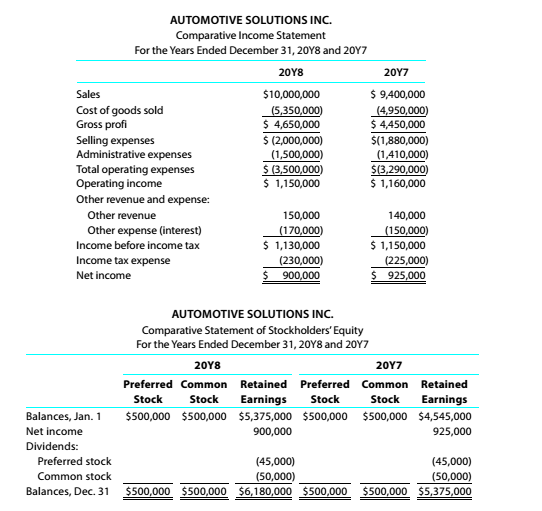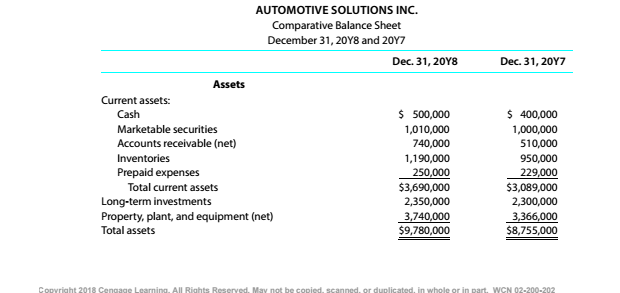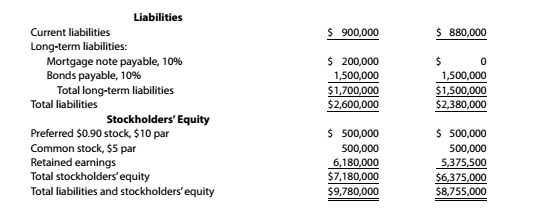Chapter 9, Problem 9.4.6P

Chapter
Section
Textbook Problem

Twenty metrics of liquidity, solvency, and profitabilityThe comparative financial statements of Automotive Solutions Inc. are as follows. The market price of Automotive Solutions Inc. common stock was $119.70 on December 31, 20Y8InstructionsInventory turnover To determine Concept Introduction: Inventory Turnover Ratio: Inventory Turnover Ratio measures the efficiency of the company in converting its inventory into sales. It is calculated by dividing the Cost of goods sold by the Average inventory. The formula of the Inventory Turnover Ratio is as follows: Inventory Turnover Ratio=Cost of goods soldAverage inventory Note: Average inventory is calculated with the help of following formula: Average inventory=(Beginning inventory + Ending inventory)2 Day's sales in inventory: Days sales in inventory represent the number of days the inventory waits for the sale. It is calculated using the following formula: Day Sales in Inventory = Inventory *365Cost of Goods Sold To Calculate: The Inventory Turnover. Explanation The Inventory Turnover is calculated as follows:  20Y8 Cost of Goods Sold (A)$ 5,350,000 Beginning Inventory (B)

Still sussing out bartleby?

Check out a sample textbook solution.

See a sample solution

The Solution to Your Study Problems

Bartleby provides explanations to thousands of textbook problems written by our experts, many with advanced degrees!

Get Started

PREFERRED STOCK RATE OF RETURN What will be the nominal rate of return on a perpetual preferred stock with a 10...

Fundamentals of Financial Management, Concise Edition (with Thomson ONE - Business School Edition, 1 term (6 months) Printed Access Card) (MindTap Course List)

What are the four phases in the typical business cycle?

Foundations of Business (MindTap Course List)

Explain how absolute advantage and comparative advantage differ.

Principles of Microeconomics (MindTap Course List)